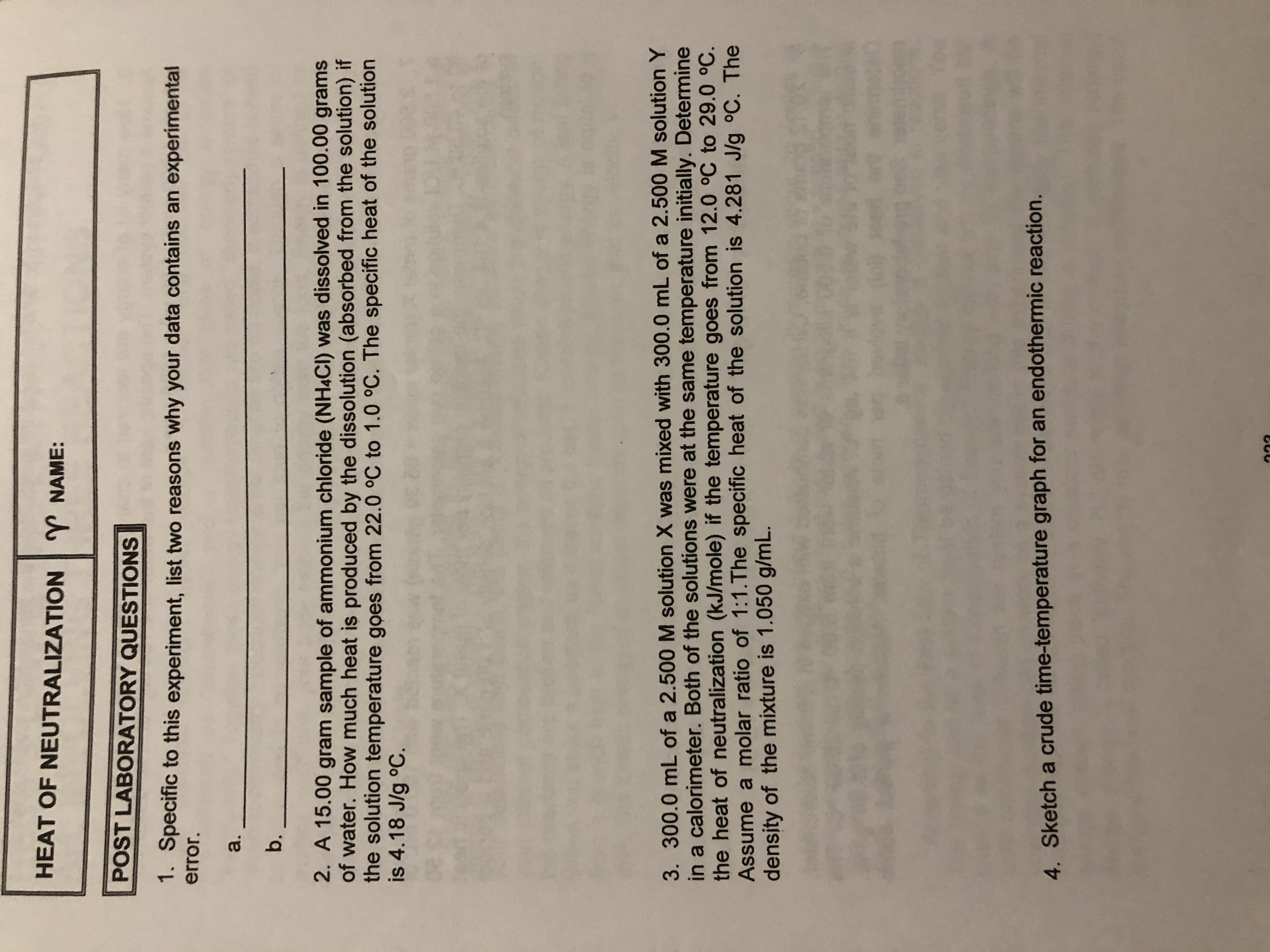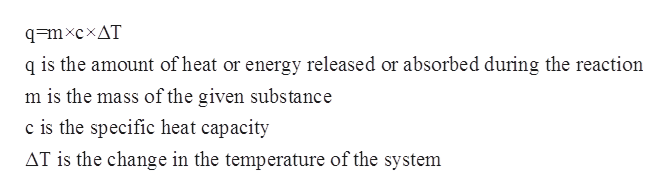# HEAT OF NEUTRALIZATIONNAME:POST LABORATORY QUESTIONS1. Specific to this experiment, list two reasons why your data contains an experimentalerror.a.b.2. A 15.00 gram sample of ammonium chloride (NH4CI) was dissolved in 100.00 gramsof water. How much heat is produced by the dissolution (absorbed from the solution) ifthe solution temperature goes from 22.0 oC to 1.0 oC. The specific heat of the solutionis 4.18 J/g °C3. 300.0 mL of a 2.500 M solution X was mixed with 300.0 mL of a 2.500 M solution Yin a calorimeter. Both of the solutions were at the same temperature initially. Determinethe heat of neutralization (kJ/mole) if the temperature goes from 12.0 °C to 29.0 °C.Assume a molar ratio of 1:1.The specific heat of the solution is 4.281 J/g °C. Thedensity of the mixture is 1.050 g/mL.4. Sketch a crude time-temperature graph for an endothermic reaction.

Question
1 views

Question 2help_outlineImage TranscriptioncloseHEAT OF NEUTRALIZATION NAME: POST LABORATORY QUESTIONS 1. Specific to this experiment, list two reasons why your data contains an experimental error. a. b. 2. A 15.00 gram sample of ammonium chloride (NH4CI) was dissolved in 100.00 grams of water. How much heat is produced by the dissolution (absorbed from the solution) if the solution temperature goes from 22.0 oC to 1.0 oC. The specific heat of the solution is 4.18 J/g °C 3. 300.0 mL of a 2.500 M solution X was mixed with 300.0 mL of a 2.500 M solution Y in a calorimeter. Both of the solutions were at the same temperature initially. Determine the heat of neutralization (kJ/mole) if the temperature goes from 12.0 °C to 29.0 °C. Assume a molar ratio of 1:1.The specific heat of the solution is 4.281 J/g °C. The density of the mixture is 1.050 g/mL. 4. Sketch a crude time-temperature graph for an endothermic reaction. fullscreen
check_circle

Step 1

The specific heat capacity for a given chemical substance or a solution refers to the amount of heat that is needed to increase the temperature of a given substance per unit of its mass. The mathematical relation between specific heat capacity, mass, change in temperature and the heat of the solution is given by the following equation:help_outlineImage Transcriptioncloseq=mxcxAT q is the amount of heat or energy released or absorbed during the reaction m is the mass of the given substance c is the specific heat capacity AT is the change in the temperature of the system fullscreen
Step 2

The initial temperature is given to be 1.0oC

The final temperature after is given to be 22.0 oC

The specific heat capacity of solution is given to be 4.18 J g-1 oC-1.

The mass of the sample is given to be 15.00 g and the mass of w...

### Want to see the full answer?

See Solution

#### Want to see this answer and more?

Solutions are written by subject experts who are available 24/7. Questions are typically answered within 1 hour.*

See Solution
*Response times may vary by subject and question.
Tagged in

### Physical Chemistry